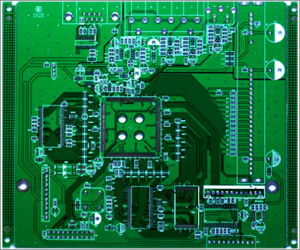# The definition of impedance PCB board

First, the printed circuit board impedance characteristics:

According to the theory of signal transmission, the signal is a function of time and distance variables, so each part of the signal may change on the line. Therefore, the AC impedance of the connection, that is, the ratio of the change of the voltage to the change of the current is determined as the characteristic impedance of the transmission line: the characteristic impedance of the transmission line is only related to the characteristics of the signal connection itself. In the actual circuit, the resistance value of the wire itself is smaller than the distributed impedance of the system. In the high-frequency circuit, the characteristic impedance mainly depends on the distributed capacitance of the unit distributed capacitance and the unit distributed inductance. The characteristic impedance of an ideal transmission line depends only on the unit distribution capacitance of the connection and the unit distribution inductance.

Second, the calculation of the characteristic impedance of the printed circuit board:

The proportional relationship between the rising edge time of the signal and the time required for the signal to be transmitted to the receiving end determines whether the signal connection is considered to be the transmission line. The specific proportional relationship can be explained by the following formula: If the length of the wire connection on the PCB is greater than l/b, the connecting wire between the signals can be regarded as the transmission line. According to the calculation formula of the equivalent impedance of the signal, the impedance of the transmission line can be expressed by the following formula: wL>>R is satisfied in the case of high frequency (tens of megahertz to several hundred megahertz) (of course, in the range where the signal frequency is greater than 109 Hz, Considering the skin effect of the signal, this relationship needs to be carefully studied). Then for a certain transmission line, its characteristic impedance is a constant. The reflection phenomenon of the signal is caused by the inconsistency between the characteristic impedance of the driving end of the signal and the transmission line and the impedance of the receiving end. For a CMOS circuit, the output impedance of the driver end of the signal is relatively small, tens of ohms. The input impedance of the receiving end is relatively large.

Third, printed circuit board characteristic impedance control:

The characteristic impedance of the conductor on the printed circuit board is an important indicator of the circuit design. Especially in the PCB design of the high frequency circuit, it must be considered whether the characteristic impedance of the wire and the characteristic impedance required by the device or signal are consistent and matched. Therefore, there are two concepts that must be noted in the reliability design of PCB design.Fourth, printed circuit board impedance control:

There are various signal transmissions in the conductors in the circuit board. When the transmission frequency must be increased, the frequency must be increased. If the circuit itself is different due to etching, laminate thickness, wire width, etc., the impedance value will be changed. Signal distortion. Therefore, the conductor on the high-speed circuit board, the impedance value should be controlled within a certain range, called “impedance control.” The factors affecting the impedance of the PCB trace are mainly the width of the copper wire, the thickness of the copper wire, the dielectric constant of the medium, the thickness of the medium, the thickness of the pad, the path of the ground line, and the trace around the trace. Therefore, when designing the PCB, the impedance of the board trace must be controlled to avoid signal reflection and other electromagnetic interference and signal integrity problems as much as possible to ensure the stability of the actual use of the PCB board. The calculation method of the microstrip line and stripline impedance on the PCB can refer to the corresponding empirical formula.

Fifth, the printed circuit board impedance matching:

In the circuit board, if there is a signal transmission, it is desirable to be smoothly transmitted to the receiving end from the emitting end of the power source with minimal energy loss, and the receiving end completely absorbs it without any reflection. To achieve this type of transmission, the impedance in the line must be equal to the impedance inside the emitting end to be called “impedance matching.” Impedance matching is one of the design elements when designing high speed PCB circuits. The impedance value has an absolute relationship with the routing method. For example, whether the distance between the surface layer (Microstrip) or the inner layer (Stripline/Double Stripline), the reference power layer or the ground layer, the trace width, and the PCB material affect the characteristic impedance of the trace. That is to say, the impedance value can be determined after wiring, and the characteristic impedance produced by different PCB manufacturers is also slightly different. General simulation software can not consider some discontinuous wiring conditions due to the limitation of the circuit model or the mathematical algorithm used. At this time, only some terminators (Temninators), such as series resistors, can be reserved on the schematic. Moderate the effect of discontinuity in the trace impedance. The only way to solve the problem is to pay attention to avoiding impedance discontinuities.